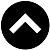-->

## MATHEMATICS FORM 1 SYLLABUS

1. Numbers
1. Base Ten Numeration
1. Identify the place value in each digit in base ten numeration
2. Read numbers in base ten numeration
3. Write numbers in base ten numeration up to one billion
4. Apply numbers in daily life
2. Natural and Whole Number
1. Distinguish between natural and whole numbers
2. Identify even ,odd, and prime numbers
3. Show even , odd and prime numbers on number lines
3. Operations with Whole Numbers
2. Subtract whole numbers
3. Multiply whole numbers
4. Divide whole numbers
5. Use the four operations in solving word problems
4. Factors And Multiples Of Numbers
1. Find factors of a number
2. Find multiples of a number
3. Use factors to find the greatest common factors(GCF) of numbers
5. Integers
1. Identify integers
3. Subtract integers
4. Multiply integers
5. Divide integers
6. Perform mixed operations on integers
2. Fractions
1. Proper, Improper and Mixed Numbers
1. Describe a fraction
2. Distinguish proper, improper fractions and mixed numbers
2. Comparison of Fractions
1. Simplify a fraction to its lowest terms
2. Identify equivalent fractions
3. Arrange fractions in order of size
3. Operations and Fractions
2. Subtract fractions
3. Multiply fractions
4. Divide fractions
5. Perform mixed operations on fractions
6. Solve word problems involving fractions
3. Decimal And Percentage
1. Decimals
1. Explain the concept of decimals
2. Convert fractions to terminating decimals and vice versa
2. Operations and Decimals
2. Subtract decimals
3. Multiply decimals
4. Perform mixed operations with decimals
5. Solve word problems involving decimals
3. Percentages
1. Express a quantity as a percentage
2. Convert a fraction into percentage and vice versa
3. Convert a decimal into percentage and vice versa
4. Apply percentages in daily life
4. Units
1. Units of Length
1. Convert one unit of length to another
2. Perform computations on metric units of length
2. Unit of Mass
1. Convert one unit of mass to another
2. Perform computation on metric units of mass
3. Units of Time
1. Convert one unit of time to another
2. Read and convert unit time of 12 hour clock to 24 hour clock and vice versa
4. Units of Capacity
1. State the standard unit of measuring capacity
2. Use the litre in daily life
5. Approximations
1. Rounding Off Numbers
1. Round off whole numbers to given place values
2. Round off decimals to a given number of decimal place
2. Significant Figures
1. Write a number to a given number of significant figures
3. Approximations in Calculations
1. Use the knowledge of rounding off of numbers in computations involving large numbers and small numbers
6. Geometry
1. Points and Lines
1. Explain the concept of a point
2. Extend the concept of a point to draw a line
3. Distinguish between a line, a line segment and a ray
2. Angles and Lines
1. Draw angles
2. Measure angles of different size using a protractor
3. Draw angles using a protractor
3. Constructions
1. Construct a perpendicular bisector to a line segment
2. Construct an angle of 60° using a pair of compasses
3. Bisect a given angle
4. Copy a given angle by construction
5. Construct parallel lines
6. Identify different types of angles formed by parallel lines and a transversal
4. Polygons And Regions
1. Describe a polygon and a region
2. Construct different types of triangles
5. Circles
1. Draw a circle
2. Describe different parts of a circle
7. Algebra
1. Algebraic Operations
1. Use symbols to form algebraic expressions
2. Simplify algebraic expressions
2. Equations with One Unknown
1. Solve an equation with one unknown
2. Form and solve an equation from word problems
3. Equations with Two Unknowns
1. Solve simultaneous equations
2. Solve linear simultaneous equations from practical situations
4. Inequalities
1. Solve linear inequalities in one unknown
2. Form linear inequalities from practical situations
8. Numbers (II)
1. Rational Numbers
1. Define a rational number
2. Perform the basic operations on rational numbers
2. Irrational Numbers
1. Define irrational numbers
3. Real Numbers
1. Define real numbers
2. Find absolute value of real numbers
3. Solve related practical problems
9. Ratio, Profit And Loss
1. Ratio
1. Express a ratio in its simplest form
2. Divide a given quantity into proportional parts
2. Profits and Loss
1. Find profit or loss
2. Calculate percentage profit and percentage profit and percentage loss
3. Simple Interest
1. Calculate simple interest
2. Solve real life problems related to simple interest
10. Coordinate Geometry
1. Coordinates of a Point
1. Read the coordinates of a point
2. Plot a point given its coordinates
3. Locate a point on the coordinates
2. Gradient (Slope) of a Line
1. Calculate the gradient of a line given two points
3. Equation of a Line
1. Find the equations of a line given the coordinates of two points on a line
4. Graphs of Linear Equations
1. Form the table of value
2. Draw the graph of a linear equation
5. Simultaneous Equations
1. Solve linear simultaneous equations graphically
11. Perimeters And Areas
1. Perimeters of Triangles and Quadrilaterals
1. Find the perimeters of triangles and quadrilaterals
2. Circumference of a Circle
1. Estimate the value of Pi ( Π)
2. Calculate the circumference of a circle
3. Areas of Rectangles and Triangles
1. Calculate the area of a rectangle
4. Areas of Trapezium and Parallelogram
1. Calculate area of a parallelogram
2. Calculate the area of a trapezium
5. Area of a Circle
1. Calculate areas of circle